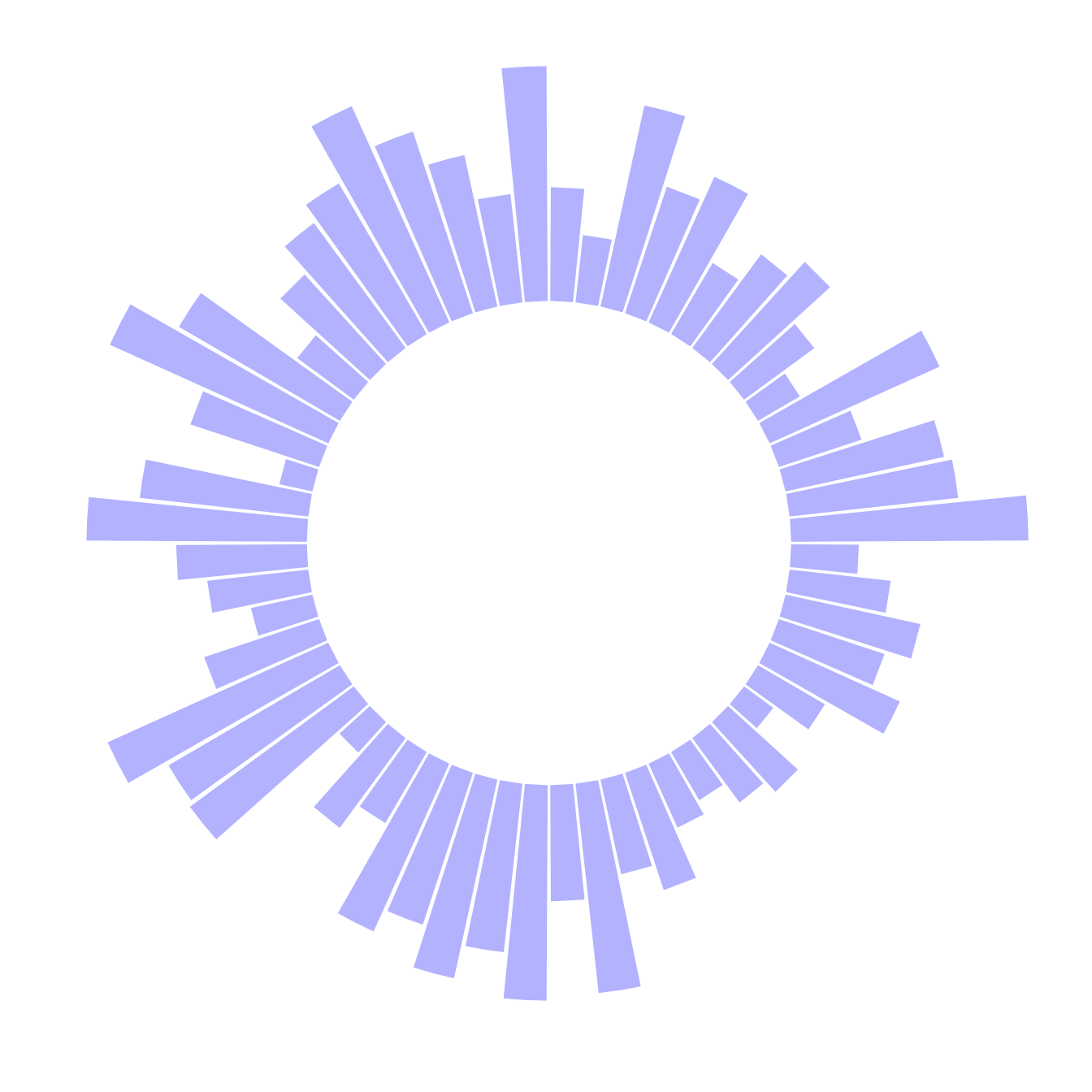# Most basic circular barplot

A circular barplot is a barplot where bars are displayed along a circle instead of a line. This post explains how to build a basic version with `R` and ggplot2. It provides the reproducible code and explain how the `coord_polar()` function works.

A circular barplot is a barplot where bars are displayed along a circle instead of a line.

The input dataset is the same than for a barplot: we need one numeric value per group (one group = one bar). (See more explanation in the barplot section).

Basically, the method is the same than to do a classic barplot. At the end, we call `coord_polar()` to make the chart circular. Note that the `ylim()` argument is really important. If it starts at 0, the bars will start from the centre of the circle. If you provide a negative value, a white circle space will appear!

This chart is not really insightful, go to the next example to learn how to add labels!``````# Libraries
library(tidyverse)

# Create dataset
data <- data.frame(
id=seq(1,60),
individual=paste( "Mister ", seq(1,60), sep=""),
value=sample( seq(10,100), 60, replace=T)
)

# Make the plot
p <- ggplot(data, aes(x=as.factor(id), y=value)) +       # Note that id is a factor. If x is numeric, there is some space between the first bar

# This add the bars with a blue color
geom_bar(stat="identity", fill=alpha("blue", 0.3)) +

# Limits of the plot = very important. The negative value controls the size of the inner circle, the positive one is useful to add size over each bar
ylim(-100,120) +

# Custom the theme: no axis title and no cartesian grid
theme_minimal() +
theme(
axis.text = element_blank(),
axis.title = element_blank(),
panel.grid = element_blank(),
plot.margin = unit(rep(-2,4), "cm")     # This remove unnecessary margin around plot
) +

# This makes the coordinate polar instead of cartesian.
coord_polar(start = 0)
p``````

# What’s next?

This chart is not really insightful, go to the next example to learn how to add labels!

Related chart types

## Contact

This document is a work by Yan Holtz. Any feedback is highly encouraged. You can fill an issue on Github, drop me a message on Twitter, or send an email pasting yan.holtz.data with gmail.com.